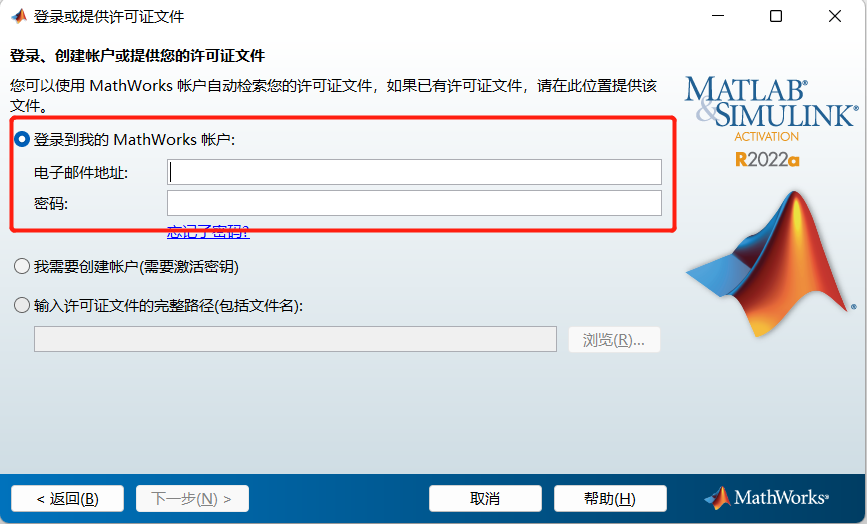MATLAB
MATLAB是美国MathWorks公司出品的商业数学软件，用于数据分析、无线通信、深度学习、图像处理与计算机视觉、信号处理、量化金融与风险管理、机器人，控制系统等领域。

# MATLAB 下载与安装

### MATLAB 下载

1.点击右上角 登录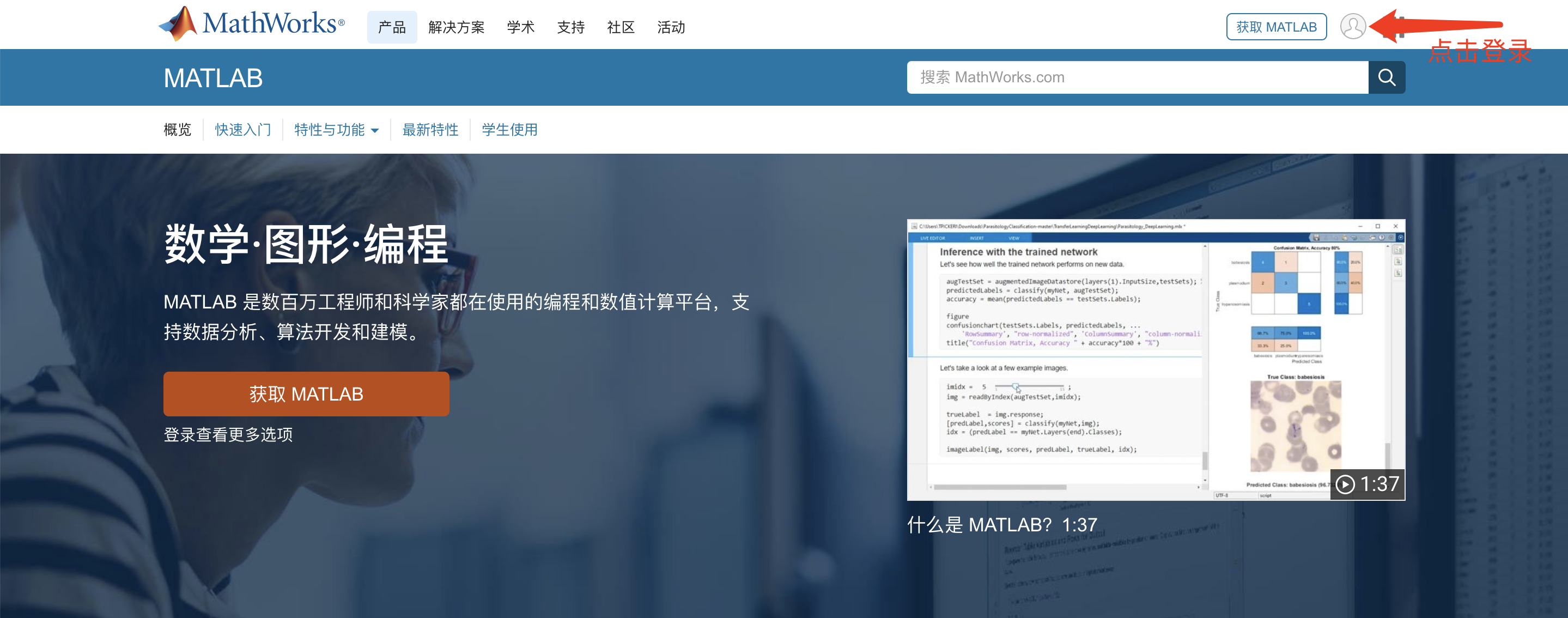2.输入正版账号与密码 进行登录3.登录成功后 点击右上角 我的账户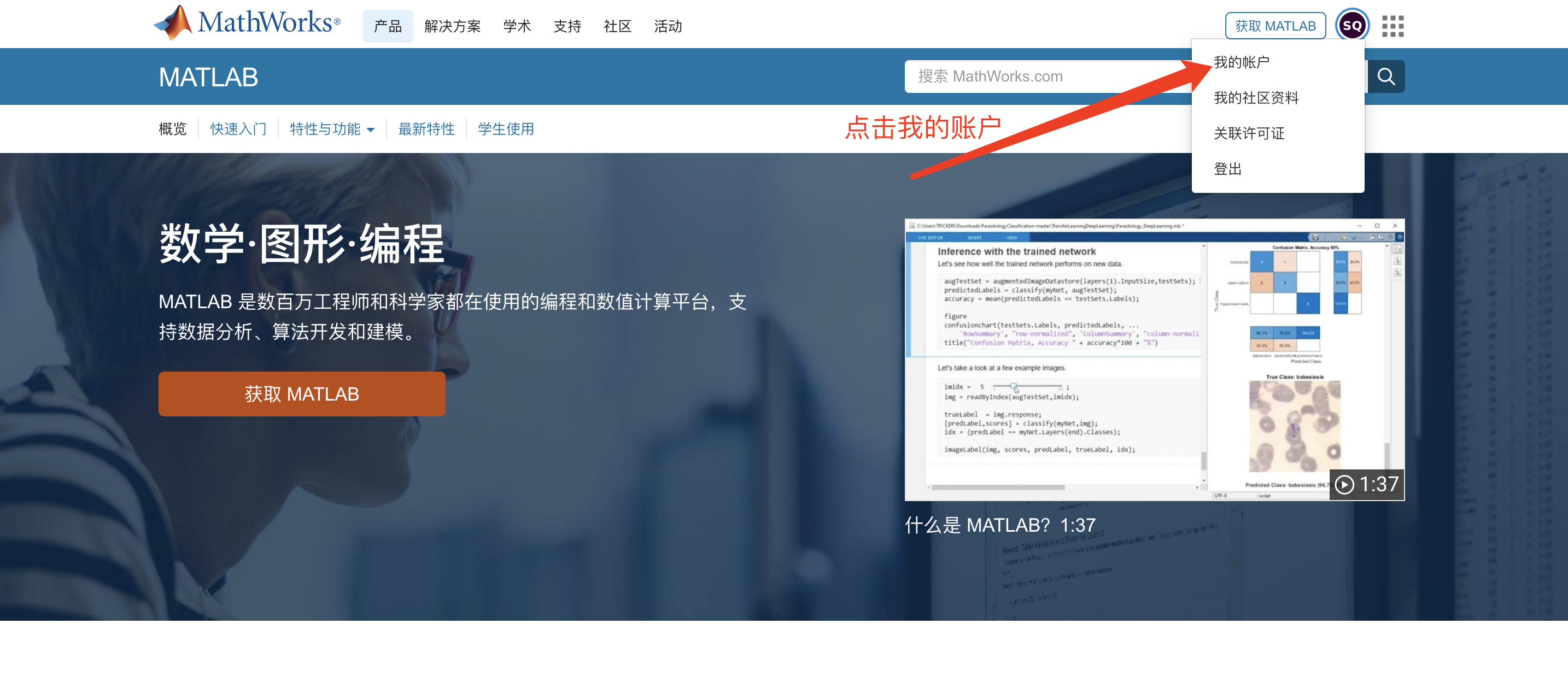4.点击我的账户页面的 下载按钮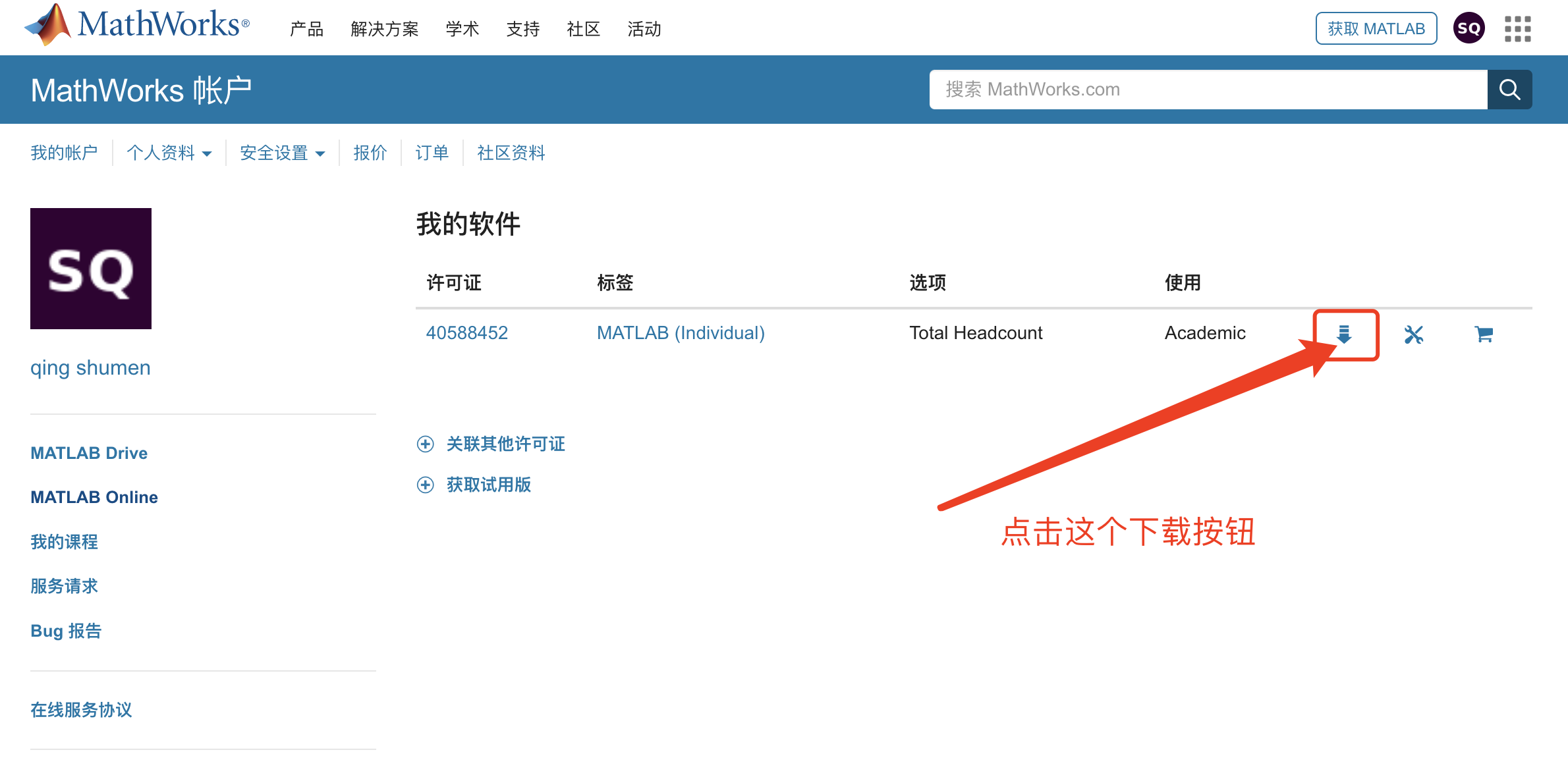5.1安装下载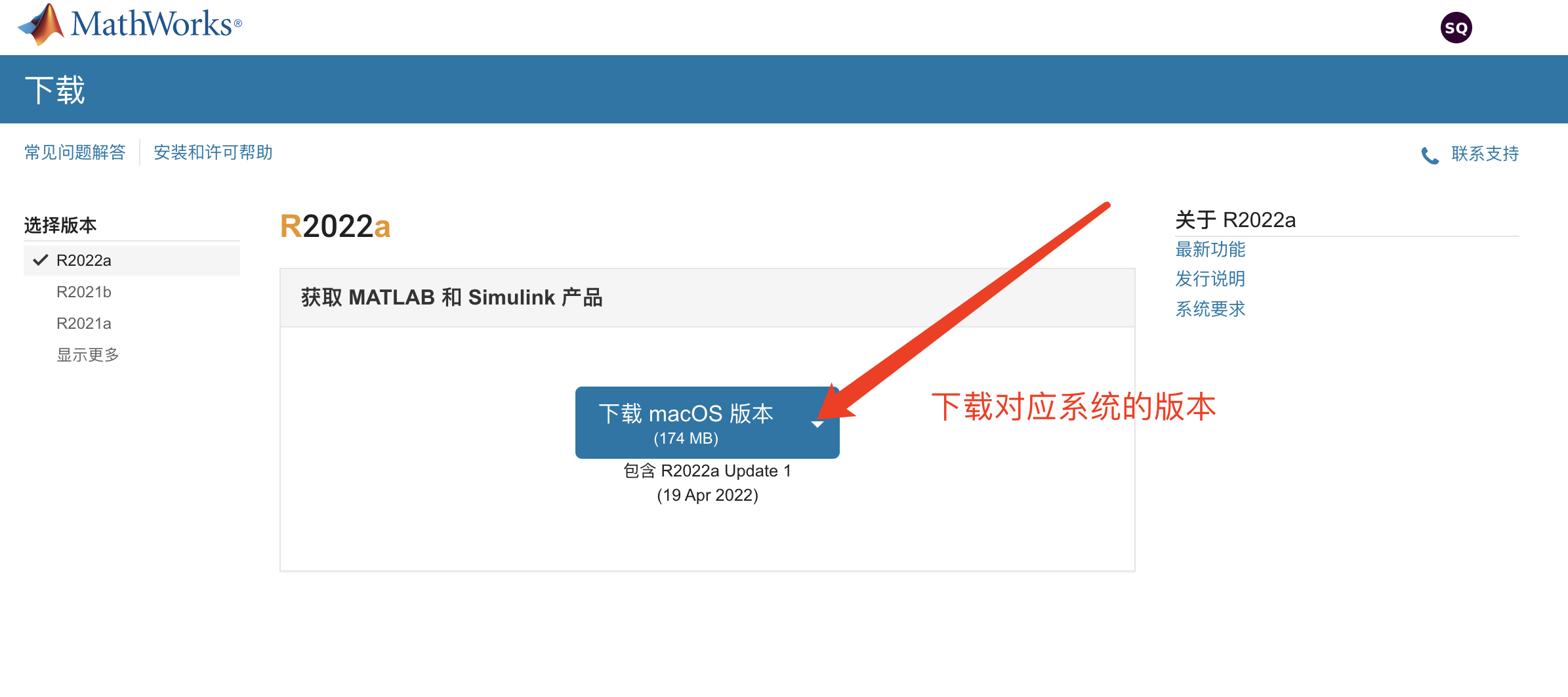5.2.安装说明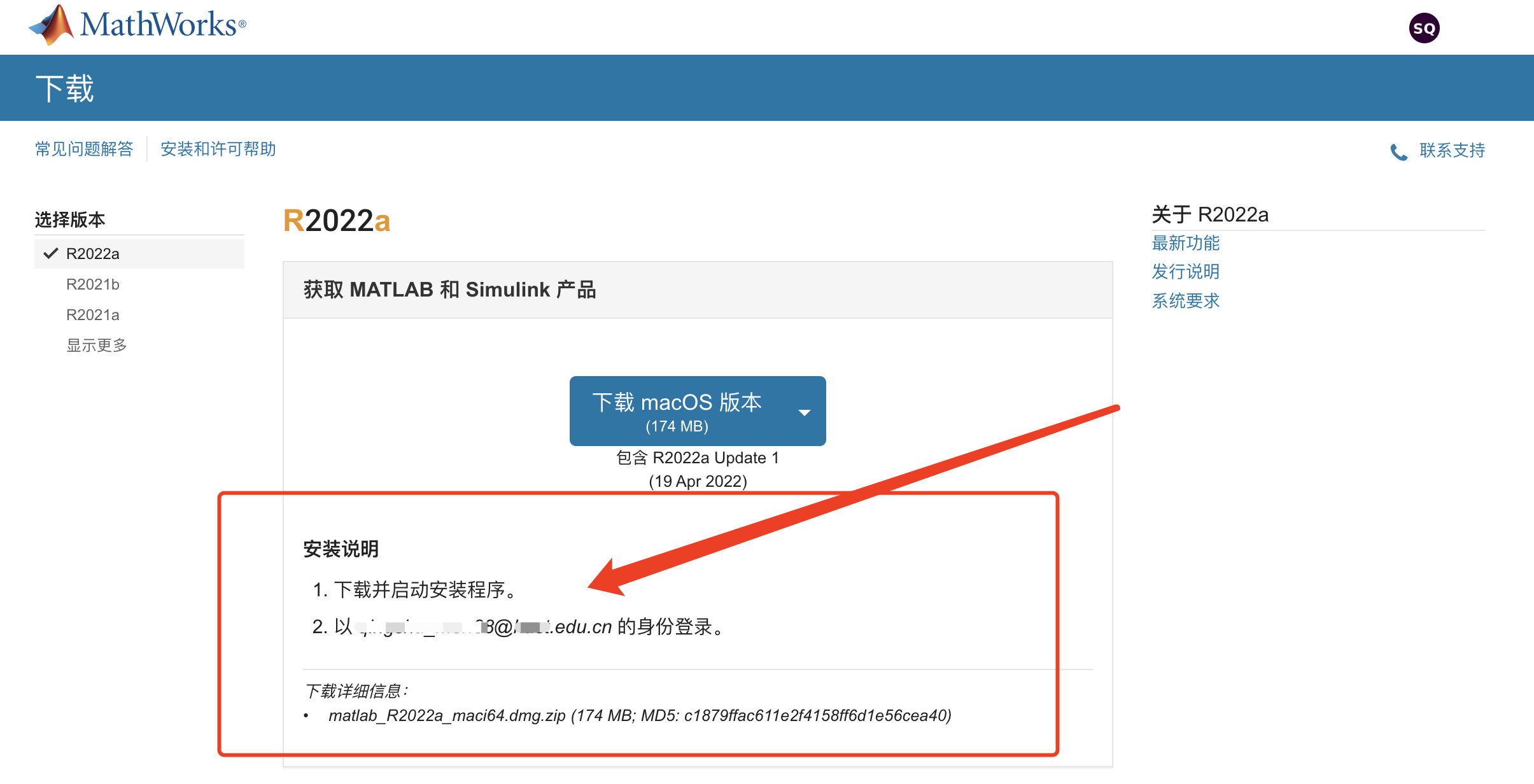6.1镜像版安装下载，右上角切换为ISO和DMG下载模式哦！（可选）6.2.镜像版安装下载说明（可选）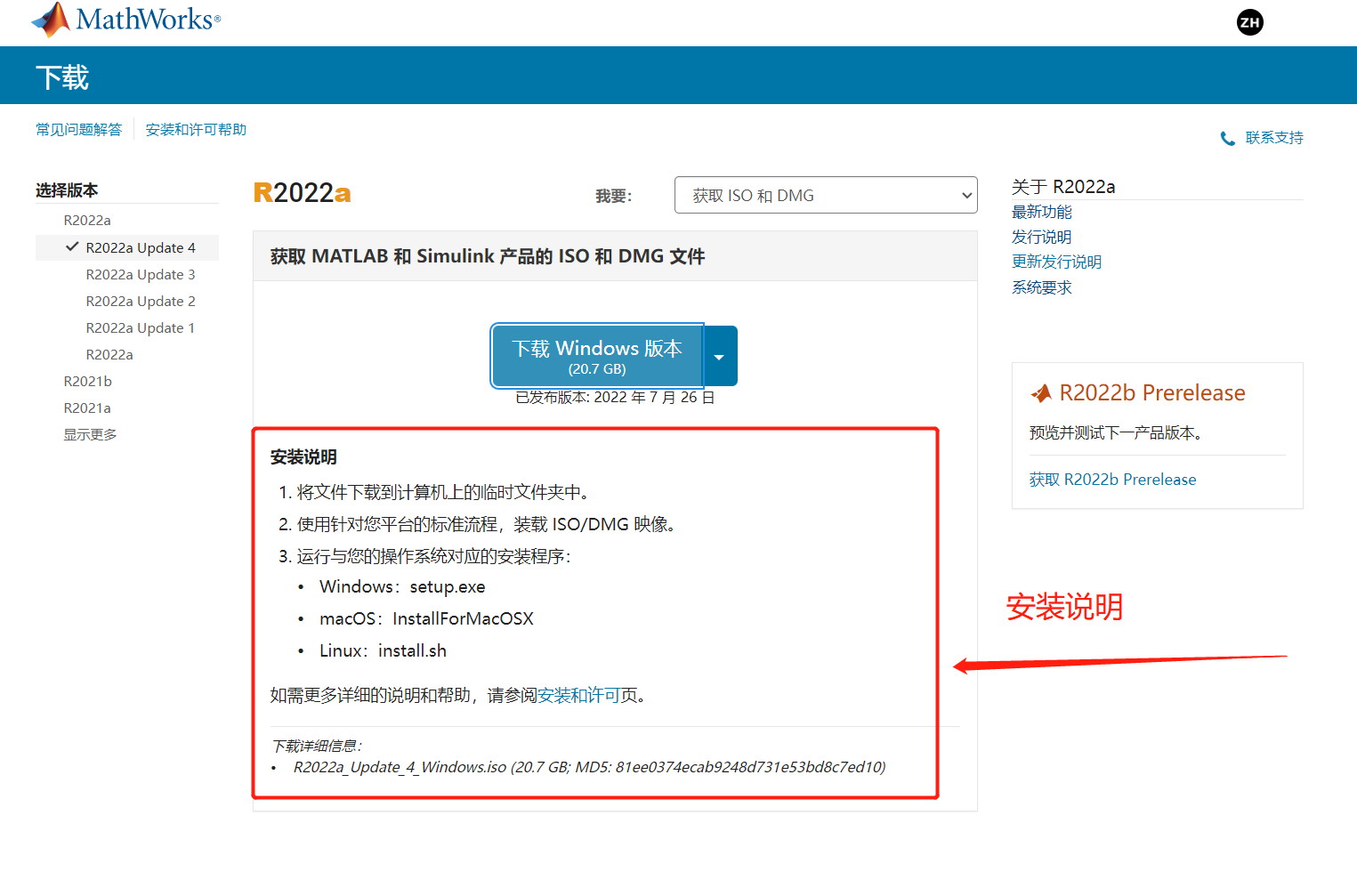### MATLAB 安装

1.1 打开安装包后，会解压一个临时文件夹 _temp_matlab_R2022a_win64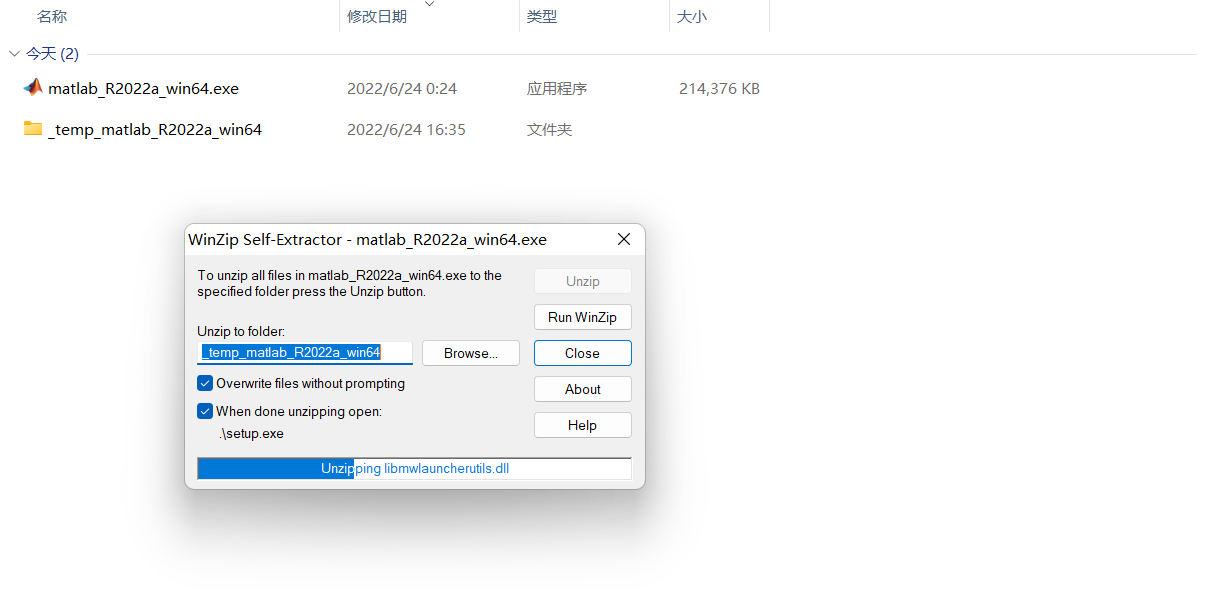1.2 第一次解压会自动打开 _temp_matlab_R2022a_win64 里面的 setup.exe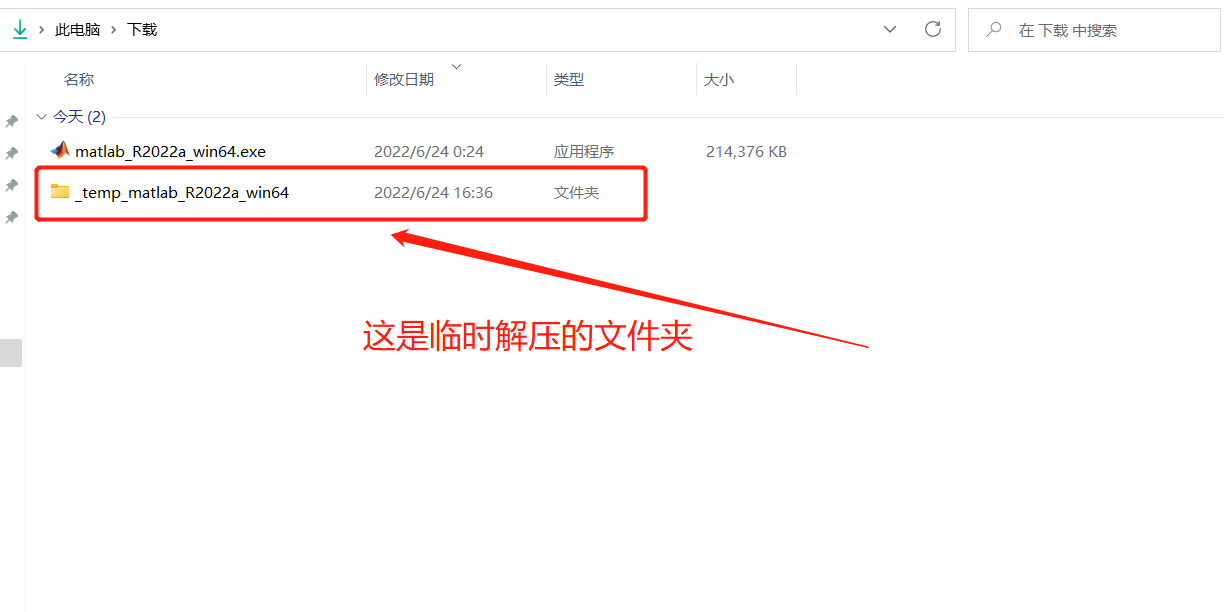1.3 正常安装登录界面，输入正版 账号密码1.4.1 如果第一次安装失败，需要重新安装的时候，只需关闭 matlab 安装程序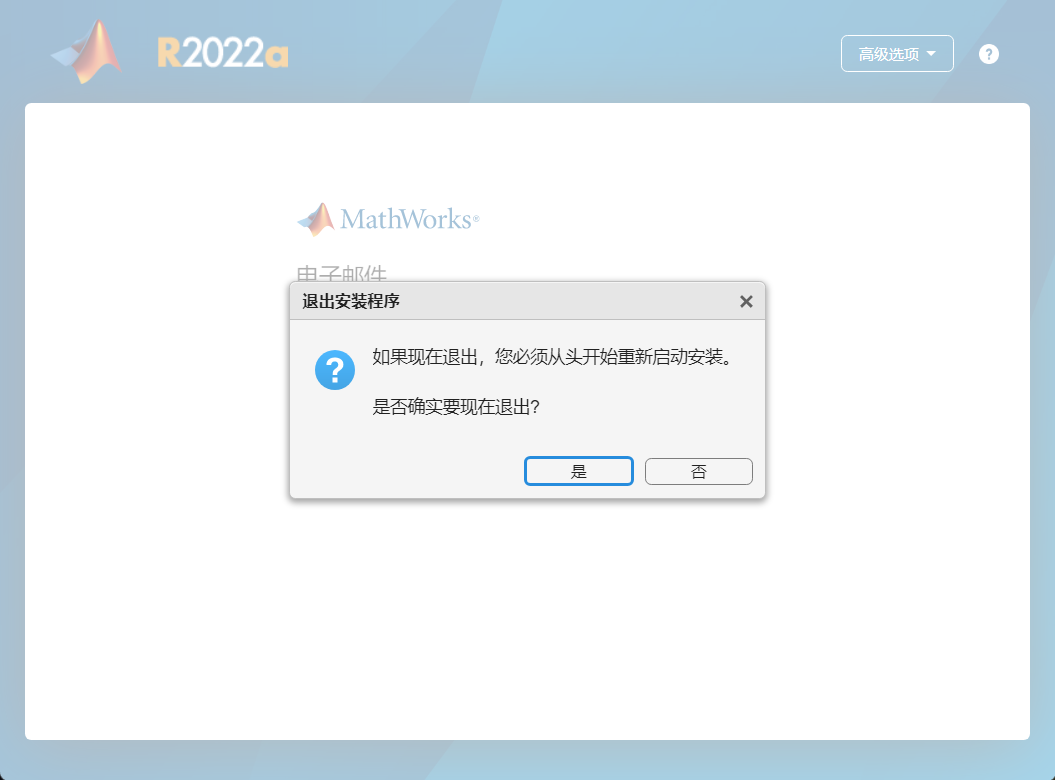1.4.2 重新打开 临时文件夹 _temp_matlab_R2022a_win64 里面的 setup.exe 重新安装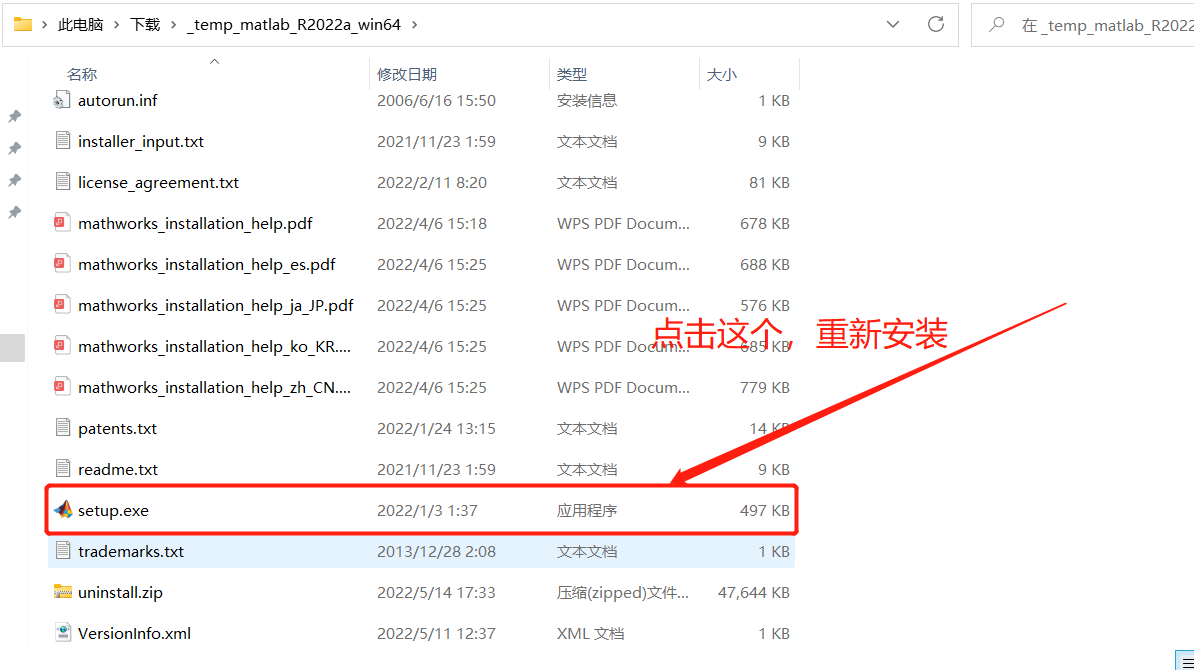1.4.2 如果仍然打开 matlab_R2022a_win64.exe 可能或出现 重复解压的 报错，建议删除临时文件夹 _temp_matlab_R2022a_win64，重新打开matlab_R2022a_win64.exe2.同意协议，下一步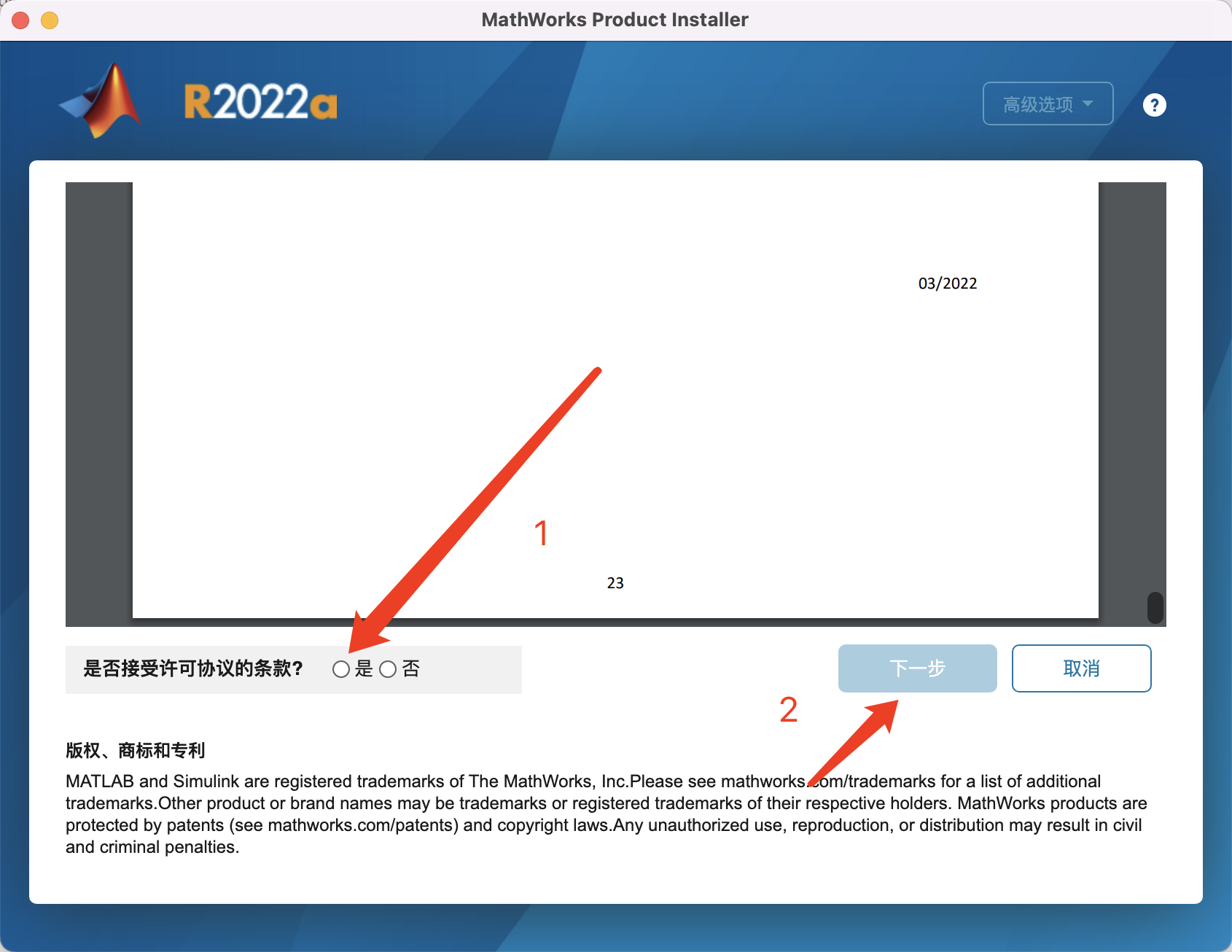3.选择许可证，点击下一步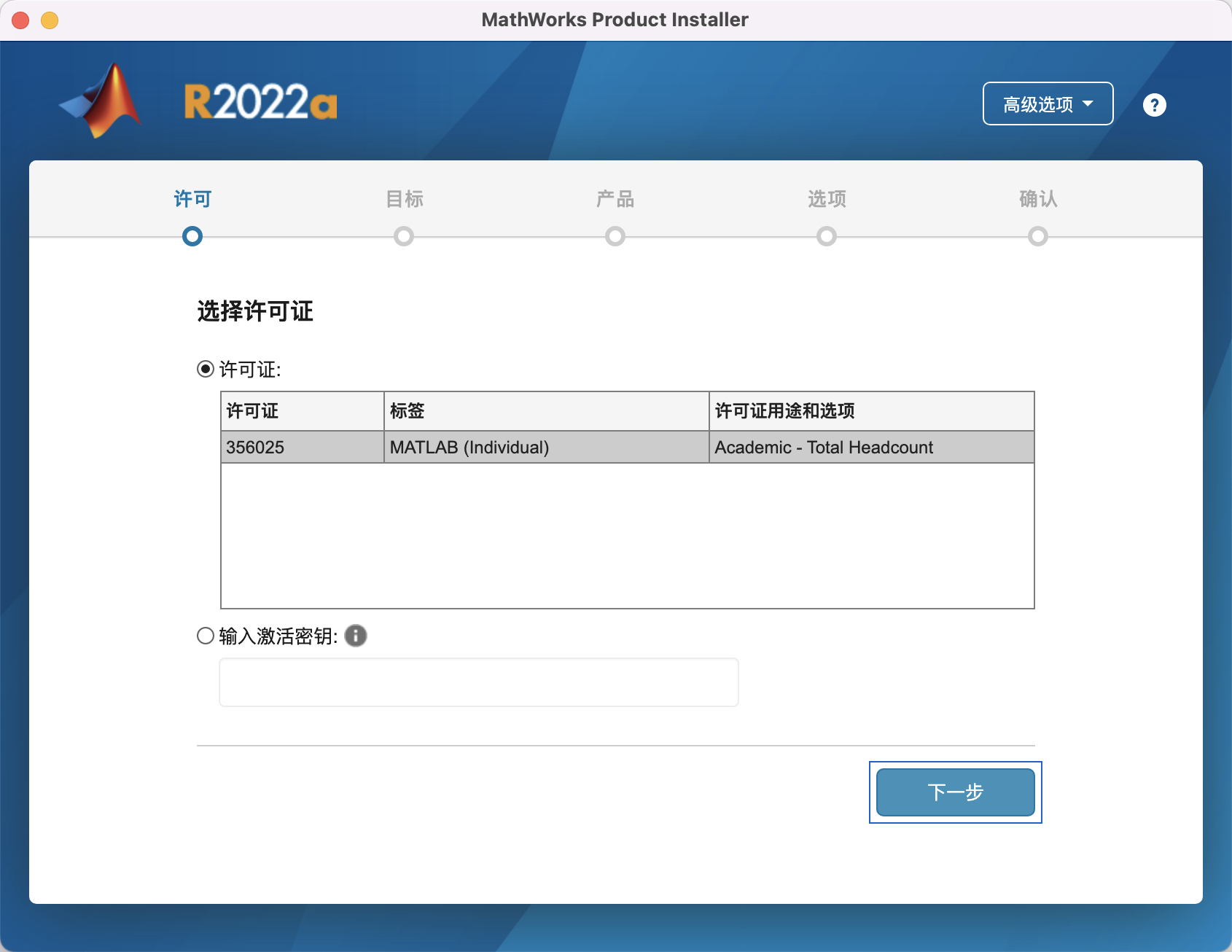1. 这里的登录名Windows 系统用户要特别注意正版系统会自动填写相应用户名默认下一步！5.选择自己需要安装的库与工具包，一般建议全选，虽然下载文件大点，但是后续省事。6.默认下一步，开始安装，等待安装成功！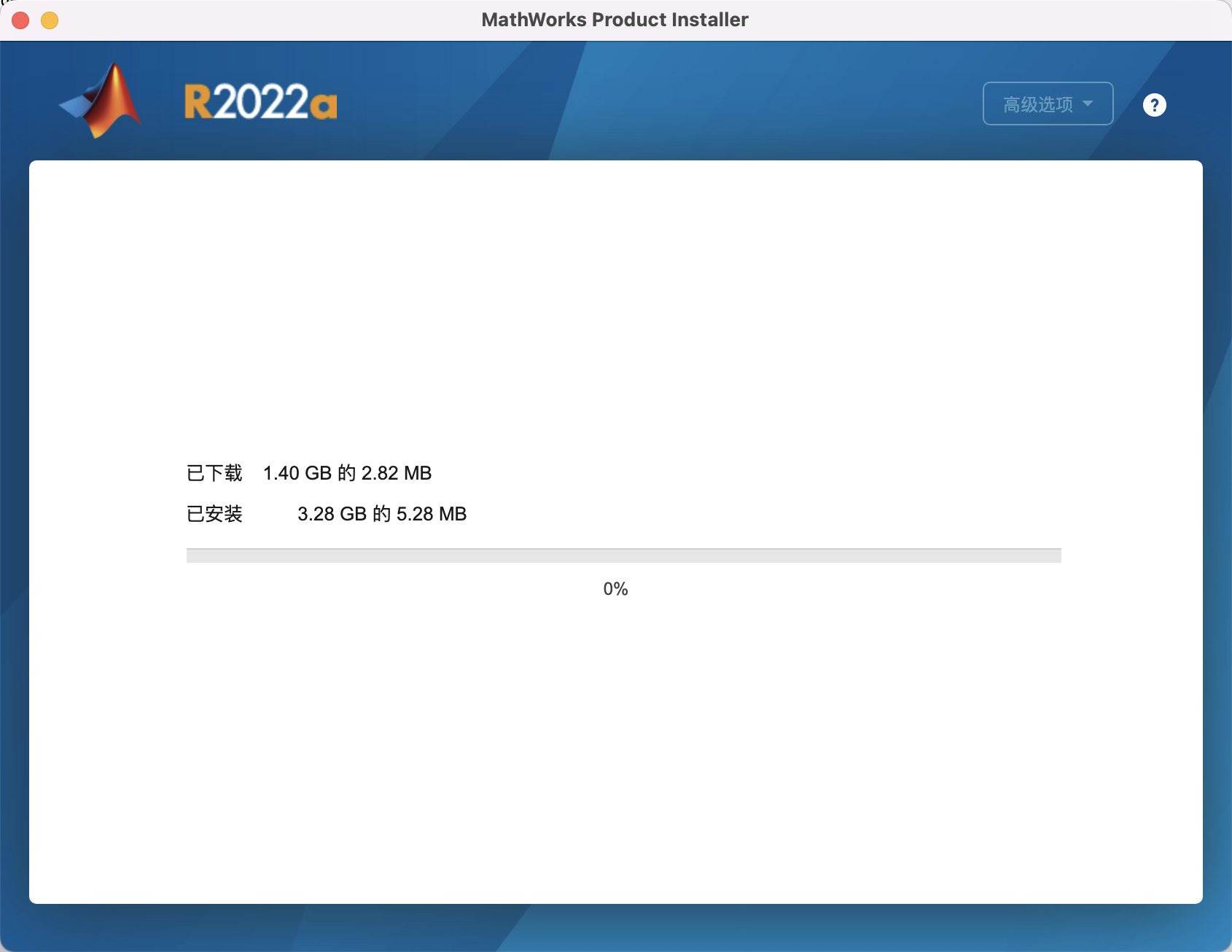7.安装成功！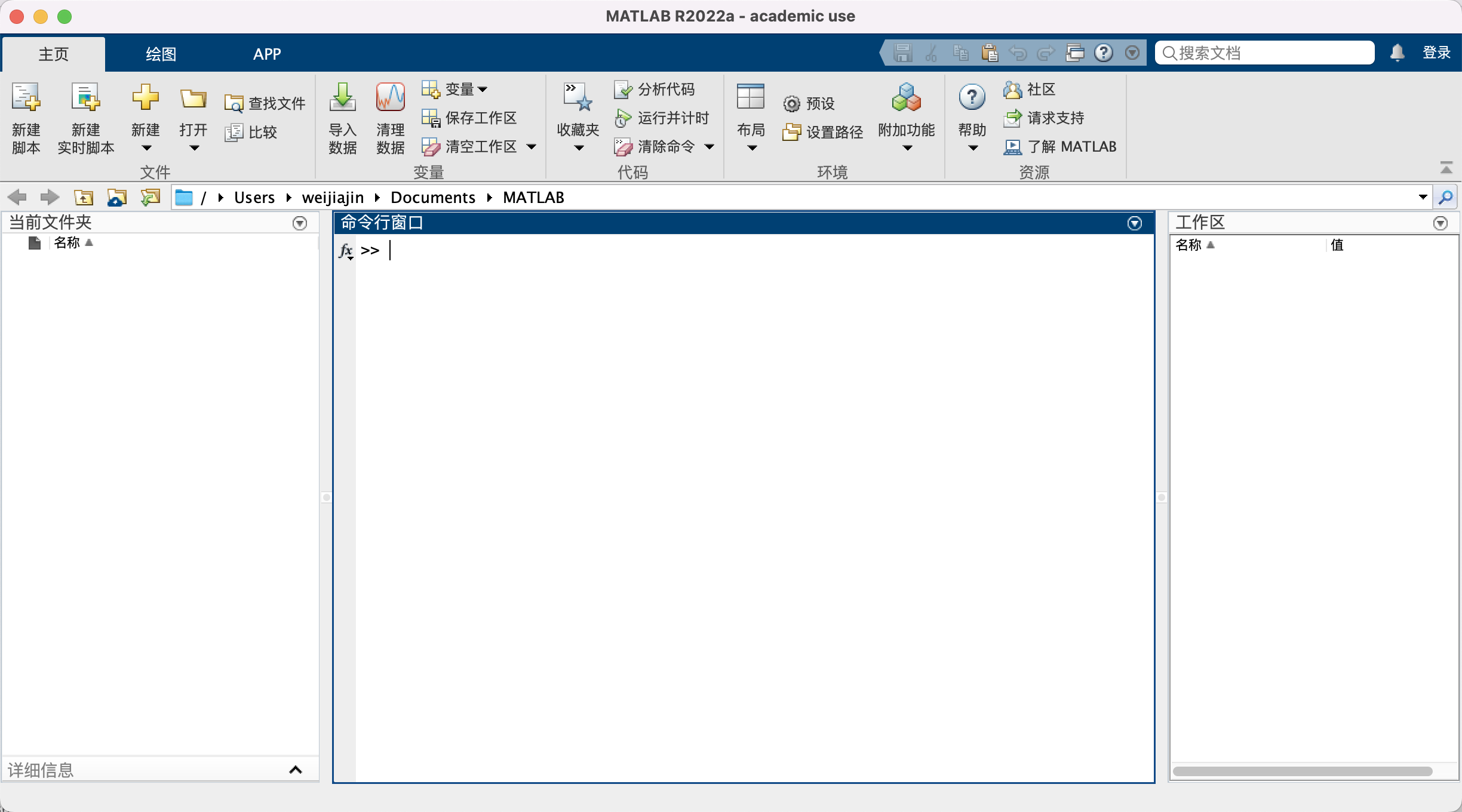### 常用界面 （可选）

1.官网软件包下载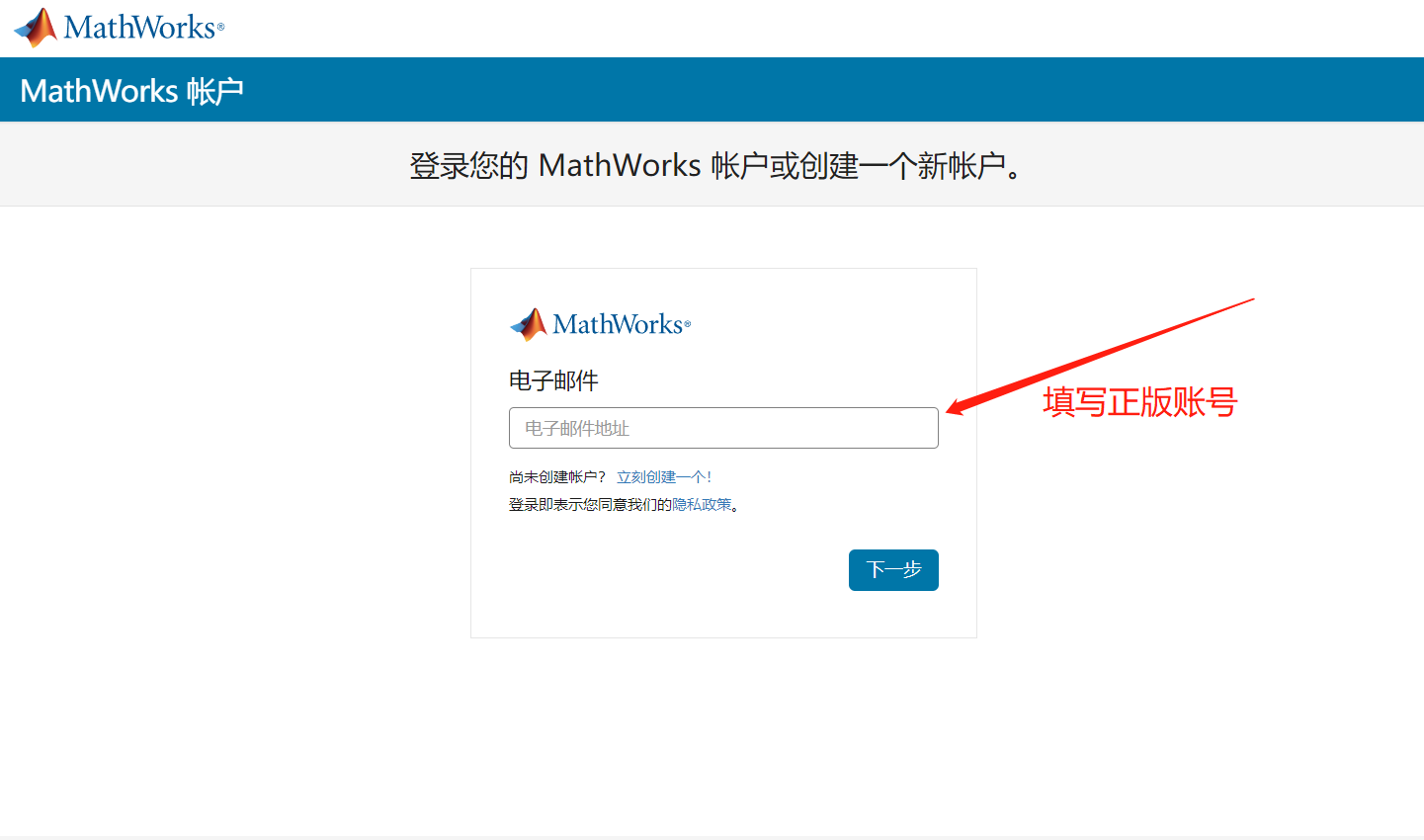1. 密码更改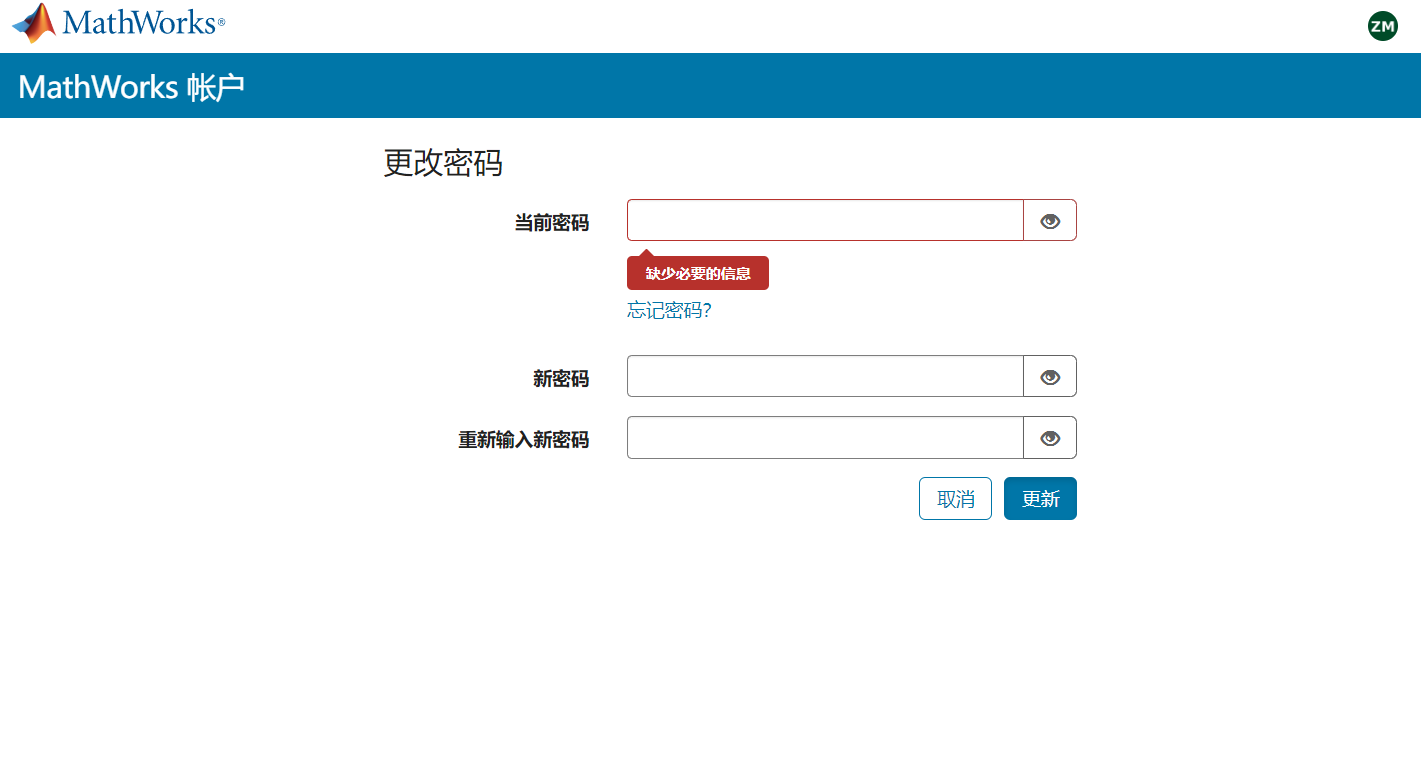3.双重验证

https://ww2.mathworks.cn/mwaccount/profiles/security/two_step_verification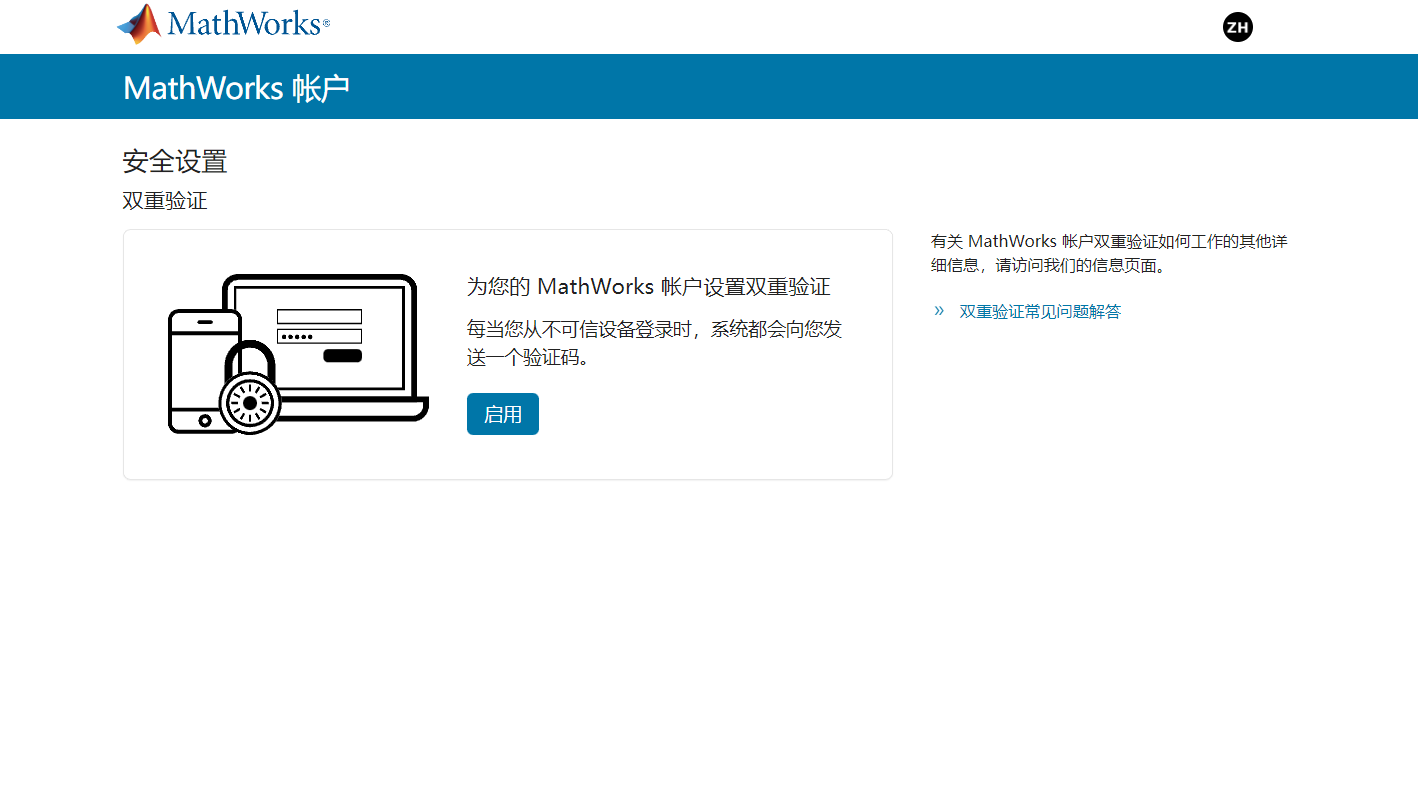1. 各个版本软件下载1. Matlab Online

https://matlab.mathworks.com/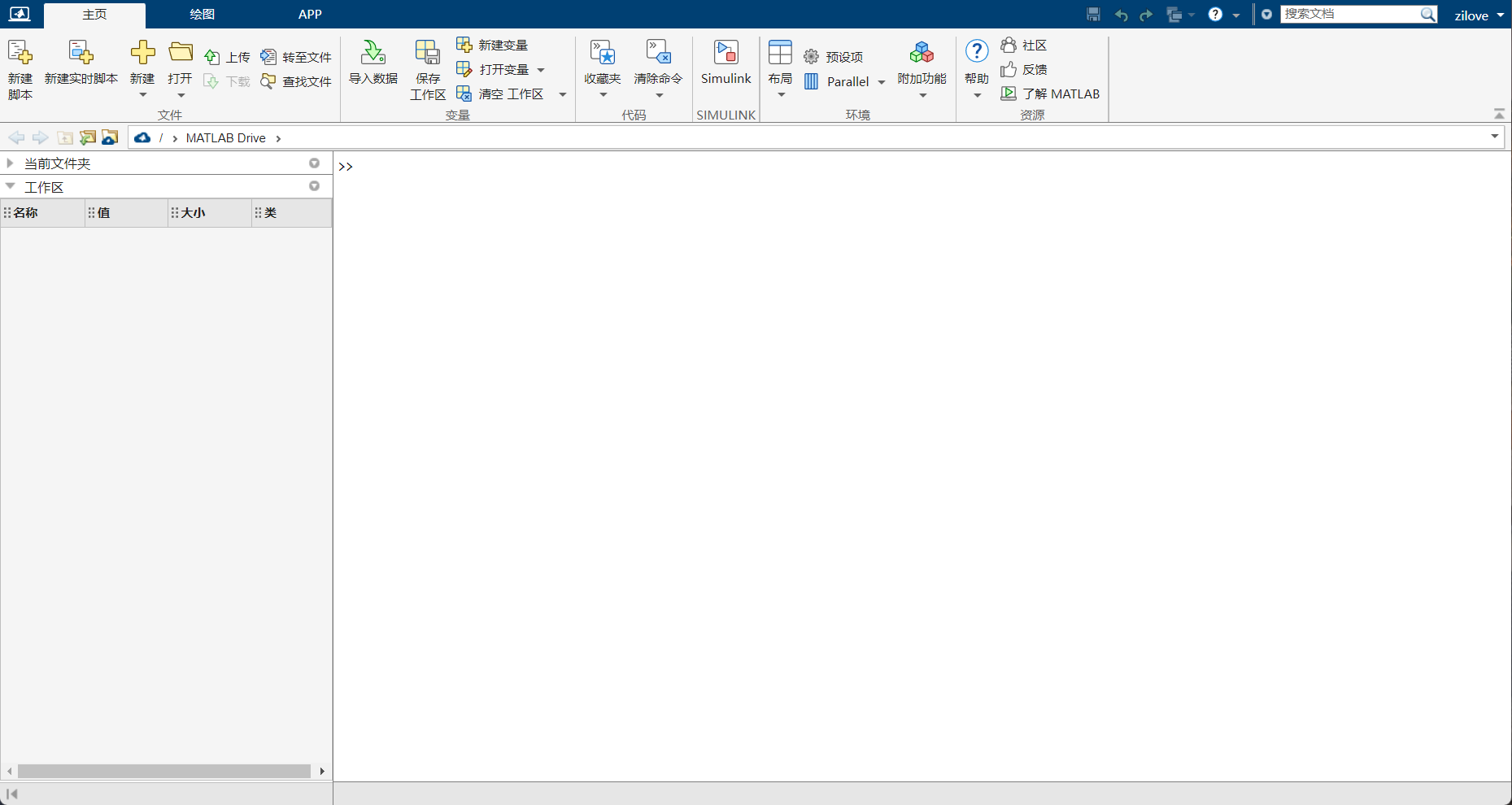### MATLAB 工具库下载（可选）

1.本地打开软件点击右上角登录我们的正版账号，点击主页，点击附加功能，点击获取附加功能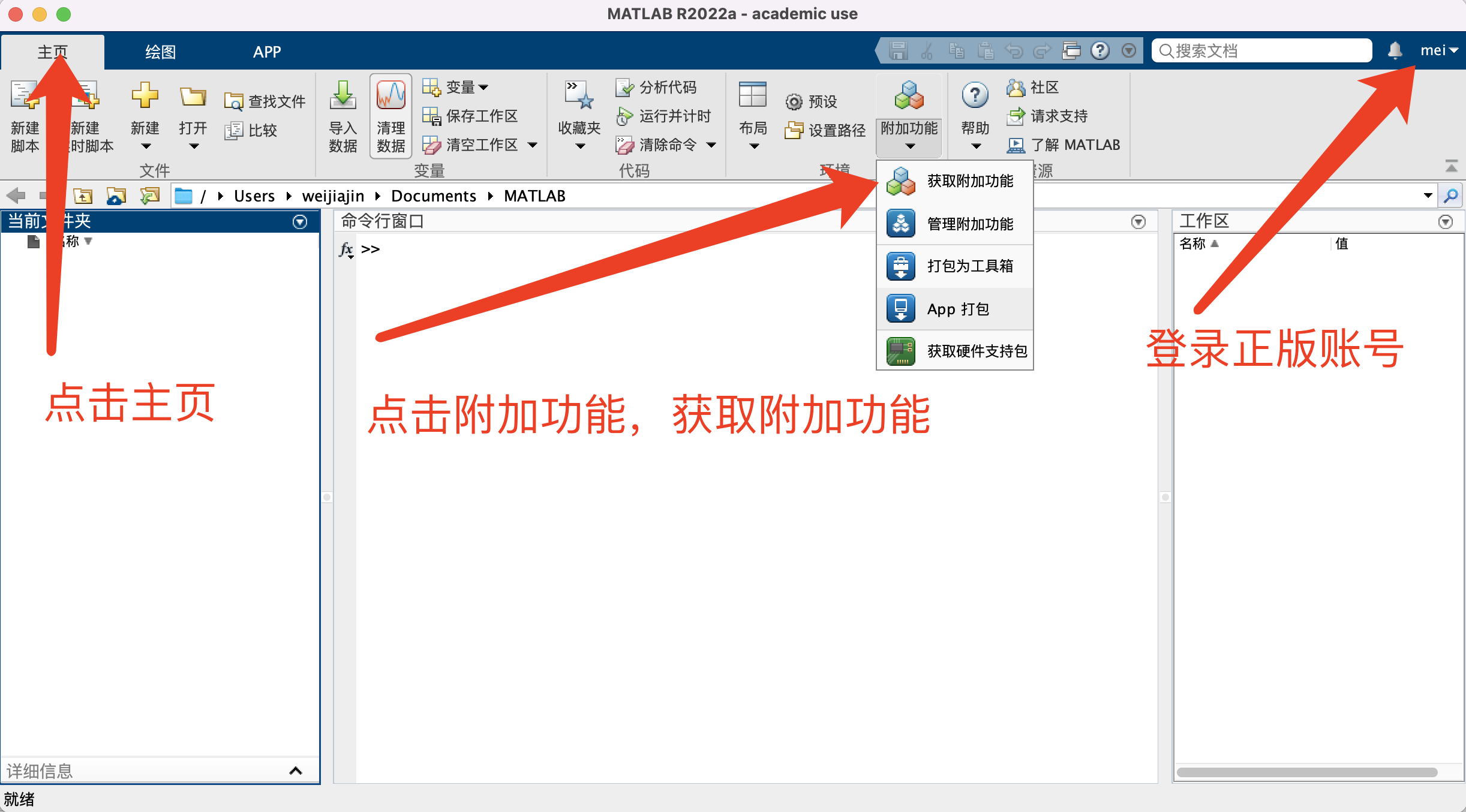2.在右上角的搜索框中搜索自己想要下载的库或者工具箱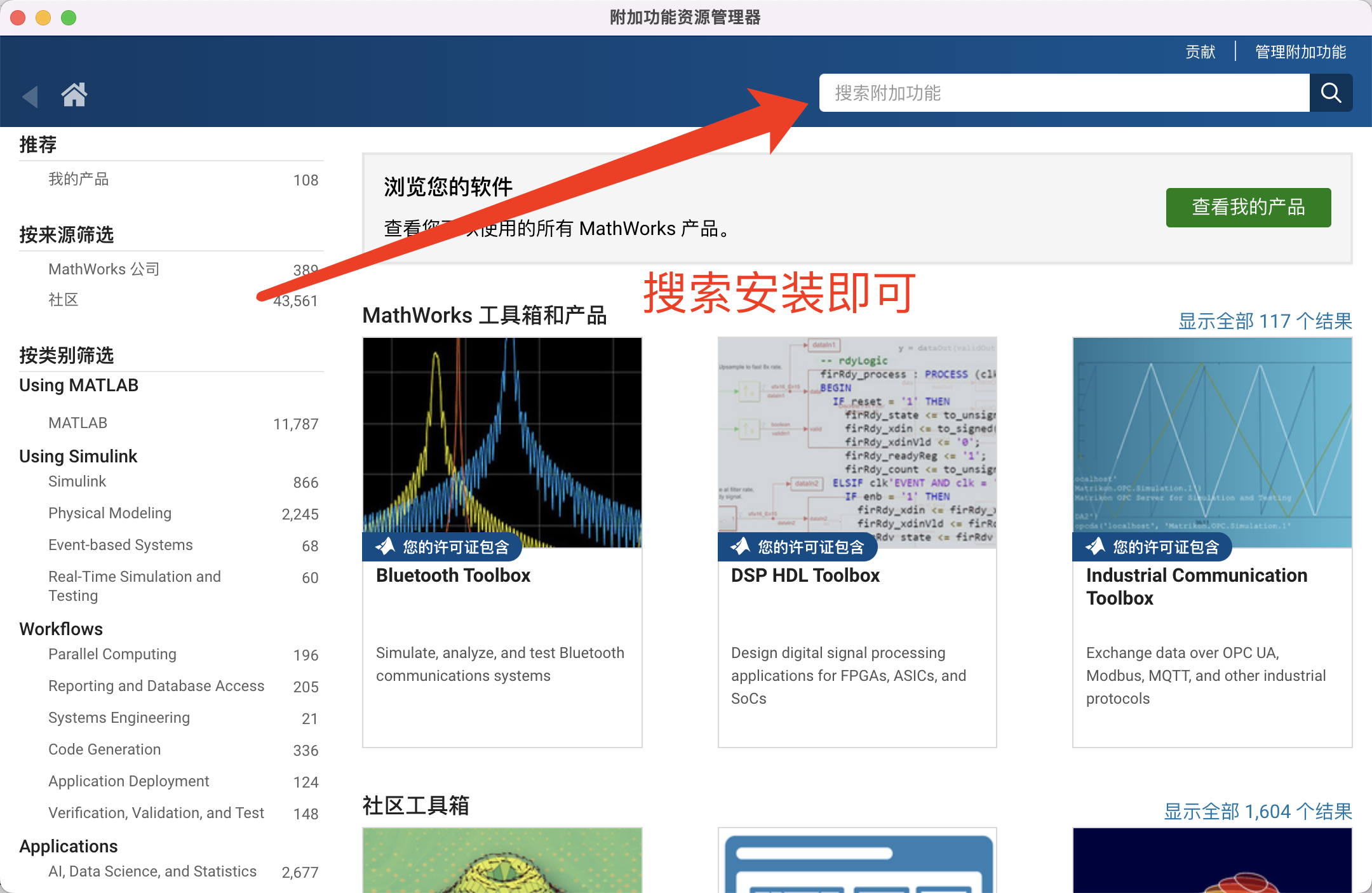### MATLAB 激活与反激活（可选）

1. 在使用我们正版账号激活之前，是要把之前电脑的所有许可证反激活的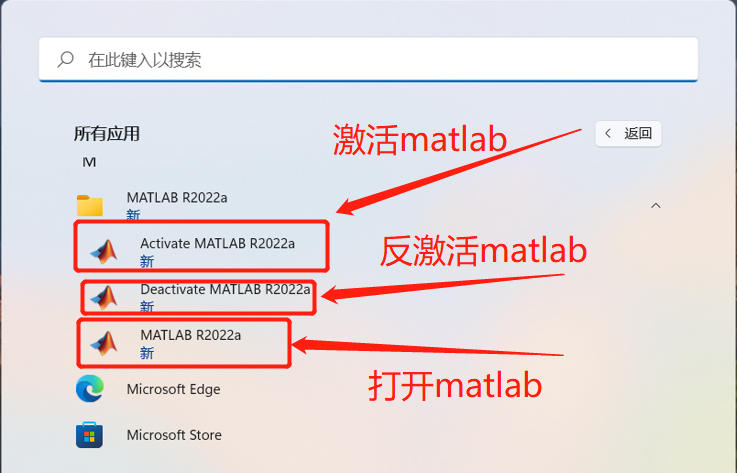1. 打开开始菜单，打开 Deactivate MATLAB R2022a1. 反激活之后，打开开始菜单，打开 Activate MATLAB R2022a